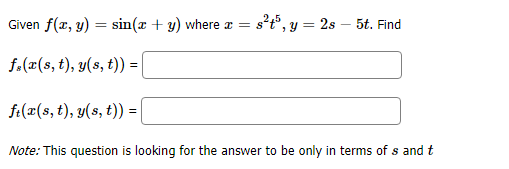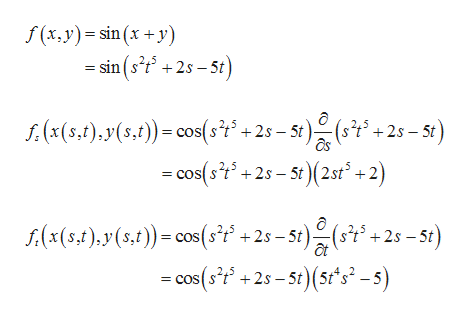# 2,5Given f(z, y)sin(x + y) where2s 5t. Findf.(ar(s, t), y(s, t))f(x(s, t), y(s, t)) =|Note: This question is looking for the answer to be only in terms of s and t

Questionhelp_outlineImage Transcriptionclose2,5 Given f(z, y) sin(x + y) where 2s 5t. Find f.(ar(s, t), y(s, t)) f(x(s, t), y(s, t)) =| Note: This question is looking for the answer to be only in terms of s and t fullscreen
check_circle

Step 1

It is given that f (x, y) = sin (x + y) where x = s2t5 and y = 2s&...help_outlineImage Transcriptionclosef(x, y) sin (x +y) = sin (s2t 2s 5t f:(x(5,t),y(s,t)) cos(st +2s-5t)(s5 - cos(st+25-5t)(2st +2) +2s 5t s(x(5,1)y(5,))cos(sf +2s-5t)(s +2s-5t) at -cos(f+2-5t)(5t*s-s) fullscreen

### Want to see the full answer?

See Solution

#### Want to see this answer and more?

Solutions are written by subject experts who are available 24/7. Questions are typically answered within 1 hour.*

See Solution
*Response times may vary by subject and question.
Tagged in

### Calculus